unwrap

Shift phase angles

Description

example

Q = unwrap(P) unwraps the radian phase angles in a vector P. Whenever the jump between consecutive angles is greater than or equal to π radians, unwrap shifts the angles by adding multiples of ±2π until the jump is less than π. If P is a matrix, unwrap operates columnwise. If P is a multidimensional array, unwrap operates on the first dimension whose size is larger than 1.

example

Q = unwrap(P,tol) compares the jump between elements of P to the jump threshold tol instead of the default value π radians. If you specify a jump threshold less than π, unwrap uses the default jump threshold π.

example

Q = unwrap(P,[],dim) unwraps along the dimension dim.

Q = unwrap(P,tol,dim) unwraps along the dimension dim using the jump threshold tol.

Examples

collapse all

Define the $x$- and $y$-coordinates of a spiral with phase angle from 0 to $6\pi$. Plot the spiral.

t = linspace(0,6*pi,201);
x = t/pi.*cos(t);
y = t/pi.*sin(t);
plot(x,y)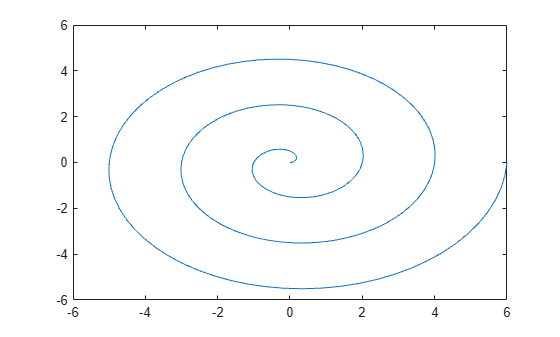Find the phase angle of the spiral from the $x$- and $y$-coordinates using the atan2 function. The atan2 function returns the angle values within the closed interval from $-\pi$ to $\pi$.

P = atan2(y,x);
plot(t,P)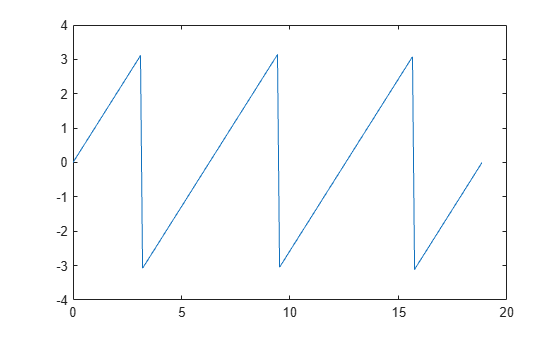Note that this plot has discontinuities. Use unwrap to eliminate the discontinuities. unwrap adds multiples of $±2\pi$ when the phase difference between consecutive elements of P are greater than or equal to the jump threshold $\pi$ radians. The shifted phase angle Q lies in the interval from 0 to $6\pi$.

Q = unwrap(P);
plot(t,Q)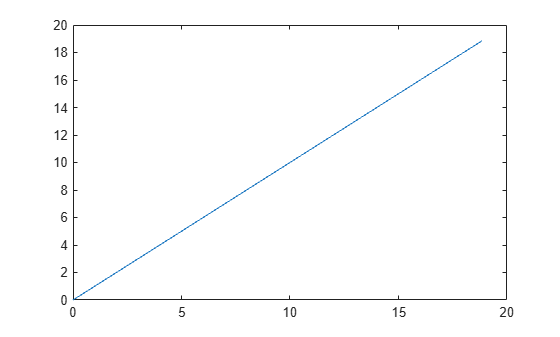Shift the phase angle of a frequency response. The phase curve has two jumps. The first jump is 3.4250 radians between W = 3 and W = 3.4, and the second jump is 6.3420 radians between W = 5 and W = 5.4. Plot the phase curve.

clear; close all;
W = [0:0.4:3, 3.4:0.4:5, 5.4:0.4:7];
P = [-1.5723
-1.5747
-1.5790
-1.5852
-1.5922
-1.6044
-1.6269
-1.6998
1.7252
1.5989
1.5916
1.5708
1.5582
-4.7838
-4.8143
-4.8456
-4.8764
-4.9002];
plot(W,P,'bo-')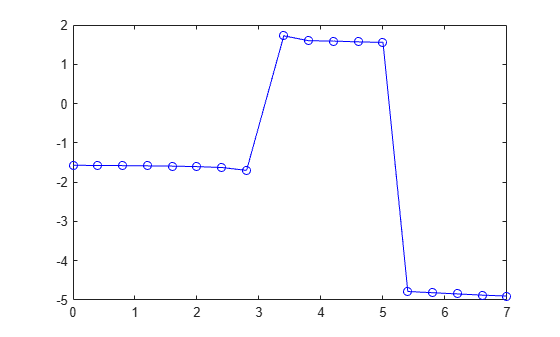Use unwrap to shift the phase angle using the default jump threshold $\pi$ radians. Plot the shifted phase curve. Both jumps are shifted since they are greater than the jump threshold $\pi$ radians.

plot(W,unwrap(P),'ro-')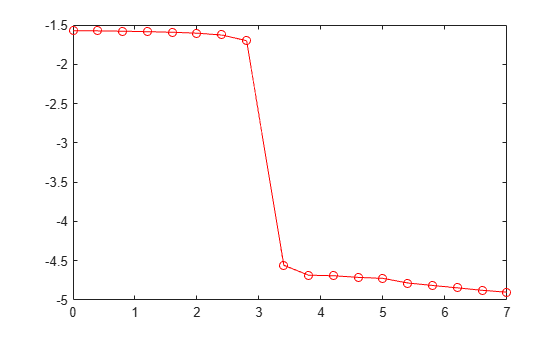Now shift the phase angle using a jump threshold of 5 radians. Plot the shifted phase curve. The first jump is not shifted since it is less than the jump threshold 5 radians.

plot(W,unwrap(P,5),'ro-')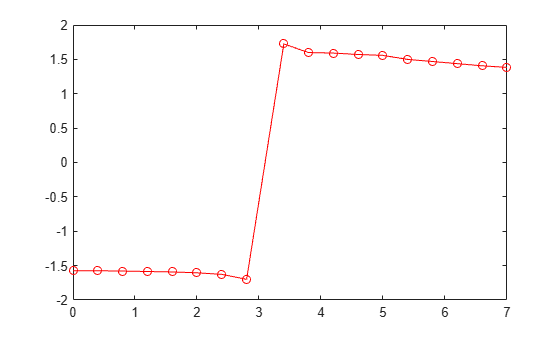Define a two-column matrix P that contains phase angles.

P = [0 7.07; 0.19 0.98; 6.67 1.18; 0.59 1.37; 0.78 1.56]
P = 5×2

0    7.0700
0.1900    0.9800
6.6700    1.1800
0.5900    1.3700
0.7800    1.5600

The phase angles P(1,2) = 7.07 and P(3,1) = 6.67 have phase differences that are larger than $\pi$ compared to the rest of the data.

Unwrap the phase angles by first comparing the elements columnwise. Specify the dim argument as 1. Use the default jump threshold $\pi$ by specifying the second argument as [].

dim = 1;
P1 = unwrap(P,[],dim)
P1 = 5×2

0    7.0700
0.1900    7.2632
0.3868    7.4632
0.5900    7.6532
0.7800    7.8432

To shift phase angles by rows instead of by columns, specify dim as 2 instead of 1.

dim = 2;
P2 = unwrap(P1,[],dim)
P2 = 5×2

0    0.7868
0.1900    0.9800
0.3868    1.1800
0.5900    1.3700
0.7800    1.5600

Input Arguments

collapse all

Input array, specified as a vector, matrix, or multidimensional array.

Data Types: single | double

Jump threshold to apply phase shift, specified as a scalar. A jump threshold less than π has the same effect as the default threshold π.

Data Types: single | double

Dimension to operate along, specified as a positive integer scalar. If no value is specified, then the default is the first array dimension whose size does not equal 1.

• unwrap(P,[],1) operates along the columns of P and returns the shifted phase angle of each column.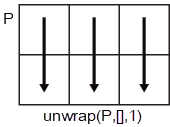• unwrap(P,[],2) operates along the rows of P and returns the shifted phase angle of each row.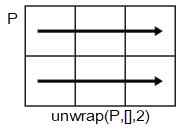If dim is greater than ndims(P), then unwrap(P,[],dim) returns P.

Data Types: single | double | int8 | int16 | int32 | int64 | uint8 | uint16 | uint32 | uint64

Output Arguments

collapse all

Shifted phase angle, returned as a vector, matrix, or multidimensional array. The size of the output Q is always the same as the size of the input P.

Data Types: single | double